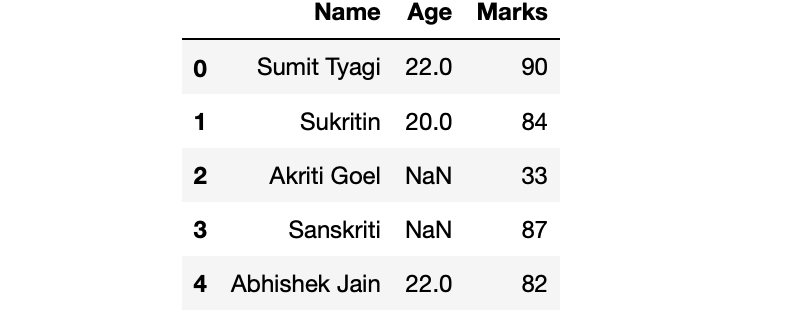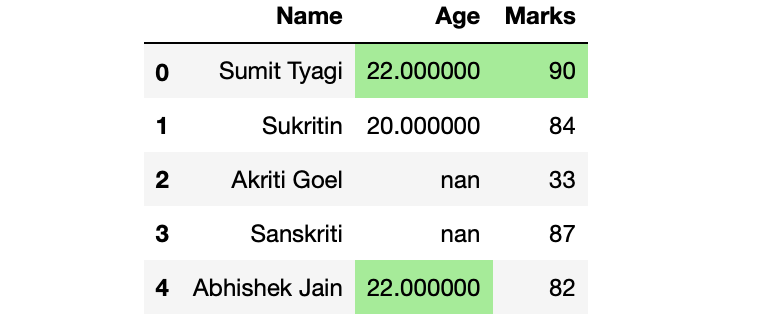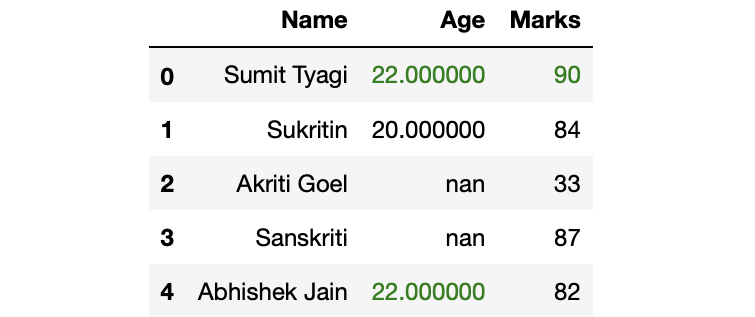Related Articles
Highlight the maximum value in last two columns in Pandas – Python
• Last Updated : 26 Jul, 2020

In this article, we will discuss how to highlight the maximum values in Pandas Dataframe. Let’s first make a dataframe:
Example:

## Python3

 `# Import Required Libraries ` `import` `pandas as pd ` `import` `numpy as np ` ` `  `# Create a dictionary for the dataframe ` `dict` `=` `{``'Name'``: [``'Sumit Tyagi'``, ``'Sukritin'``, ``'Akriti Goel'``, ` `                 ``'Sanskriti'``, ``'Abhishek Jain'``], ` `        ``'Age'``: [``22``, ``20``, np.nan, np.nan, ``22``], ` `        ``'Marks'``: [``90``, ``84``, ``33``, ``87``, ``82``]} ` ` `  `# Converting Dictionary to Pandas Dataframe ` `df ``=` `pd.DataFrame(``dict``) ` ` `  `# Print Dataframe ` `df`

Output:Now, come to the highlighting part. Our objective is to highlight cells with maximum values in last 2 columns.

#### Method 1: Highlighting Cell with maximum value in last 2 columns

We will do this by using the highlight_max() method of DataFrame property. highlight_max() method takes 3 arguments,

• subset: name of the columns of which you want to find the maximum
• color: name of the color with which you want to highlight the cell
• axis: (0/1) based on which axis you want to find the maximum.

Example:

## Python3

 `# Highlighting the maximum values  ` `# of last 2 columns ` `df.style.highlight_max(subset ``=` `[``'Age'``, ``'Marks'``], ` `                       ``color ``=` `'lightgreen'``, axis ``=` `0``)`

Output:Example:

## Python3

 `# Highlighting the maximum values of ` `# last 2 columns ` `df.style.highlight_max(subset ``=` `df.columns[``-``2``:], ` `                       ``color ``=` `'lightgreen'``, axis ``=` `0``)`

Output:## Python3

 `# Defining custom function which returns ` `# the list for df.style.apply() method ` `def` `highlight_max(s): ` `    ``is_max ``=` `s ``=``=` `s.``max``() ` `    ``return` `[``'color: green'` `if` `cell ``else` `'' ``for` `cell ``in` `is_max] ` ` `  `df.style.``apply``(highlight_max, subset ``=` `df.columns[``-``2``:])`

Output:## Python3

 `# Defining custom function which returns ` `# the list for df.style.apply() method ` `def` `highlight_max(s): ` `    ``is_max ``=` `s ``=``=` `s.``max``() ` `    ``return` `[``'background: lightgreen'` `if` `cell ``else` `'' ``for` `cell ``in` `is_max] ` ` `  `df.style.``apply``(highlight_max, subset ``=` `df.columns[``-``2``:])`

Output:Attention geek! Strengthen your foundations with the Python Programming Foundation Course and learn the basics.

To begin with, your interview preparations Enhance your Data Structures concepts with the Python DS Course.

My Personal Notes arrow_drop_up
Recommended Articles
Page :